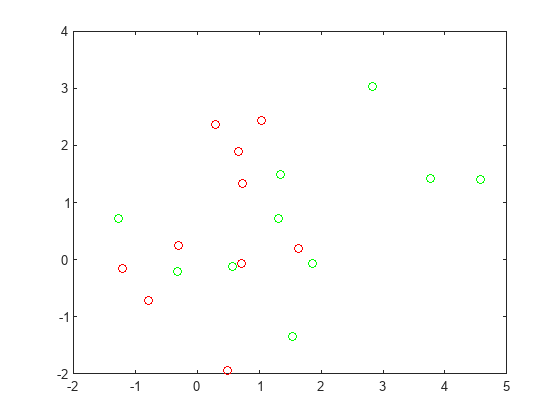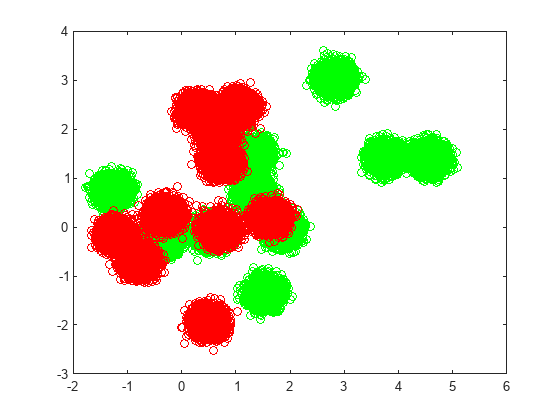Main Content

# Train Classification Ensemble in Parallel

This example shows how to train a classification ensemble in parallel. The model has ten red and ten green base locations, and red and green populations that are normally distributed and centered at the base locations. The objective is to classify points based on their locations. These classifications are ambiguous because some base locations are near the locations of the other color.

Create and plot ten base locations of each color.

```rng default % For reproducibility grnpop = mvnrnd([1,0],eye(2),10); redpop = mvnrnd([0,1],eye(2),10); plot(grnpop(:,1),grnpop(:,2),'go') hold on plot(redpop(:,1),redpop(:,2),'ro') hold off```Create 40,000 points of each color centered on random base points.

```N = 40000; redpts = zeros(N,2);grnpts = redpts; for i = 1:N grnpts(i,:) = mvnrnd(grnpop(randi(10),:),eye(2)*0.02); redpts(i,:) = mvnrnd(redpop(randi(10),:),eye(2)*0.02); end figure plot(grnpts(:,1),grnpts(:,2),'go') hold on plot(redpts(:,1),redpts(:,2),'ro') hold off``````cdata = [grnpts;redpts]; grp = ones(2*N,1); % Green label 1, red label -1 grp(N+1:2*N) = -1;```

Fit a bagged classification ensemble to the data. For comparison with parallel training, fit the ensemble in serial and return the training time.

```tic mdl = fitcensemble(cdata,grp,'Method','Bag'); stime = toc```
```stime = 9.0782 ```

Evaluate the out-of-bag loss for the fitted model.

`myerr = oobLoss(mdl)`
```myerr = 0.0572 ```

Create a bagged classification model in parallel, using a reproducible tree template and parallel substreams. You can create a parallel pool on a cluster or a parallel pool of thread workers on your local machine. To choose the appropriate parallel environment, see Choose Between Thread-Based and Process-Based Environments.

`parpool`
```Starting parallel pool (parpool) using the 'local' profile ... Connected to the parallel pool (number of workers: 6). ```
```ans = ProcessPool with properties: Connected: true NumWorkers: 6 Cluster: local AttachedFiles: {} AutoAddClientPath: true IdleTimeout: 30 minutes (30 minutes remaining) SpmdEnabled: true ```
```s = RandStream('mrg32k3a'); options = statset("UseParallel",true,"UseSubstreams",true,"Streams",s); t = templateTree("Reproducible",true); tic mdl2 = fitcensemble(cdata,grp,'Method','Bag','Learners',t,'Options',options); ptime = toc```
```ptime = 6.2527 ```

On this six-core system, the training process in parallel is faster.

`speedup = stime/ptime`
```speedup = 1.4519 ```

Evaluate the out-of-bag loss for this model.

`myerr2 = oobLoss(mdl2)`
```myerr2 = 0.0577 ```

The error rate is similar to the rate of the first model.

To demonstrate the reproducibility of the model, reset the random number stream and fit the model again.

```reset(s); tic mdl2 = fitcensemble(cdata,grp,'Method','Bag','Learners',t,'Options',options); toc```
```Elapsed time is 3.953355 seconds. ```

Check that the loss is the same as the previous loss.

`myerr2 = oobLoss(mdl2)`
```myerr2 = 0.0577 ```

Download ebook